GeeksforGeeks App
Open AppBrowser
Continue

# Perl Tutorial – Learn Perl With Examples

Perl is a general-purpose, high level interpreted and dynamic programming language. At the beginning level, Perl was developed only for system management and text handling but in later versions, Perl got the ability to handle regular expressions, and network sockets, etc. At present Perl is popular for its ability to handling the Regex(Regular Expressions). The first version of Perl was 1.0 which was released on December 18, 1987. Perl 6 is different from Perl 5 because it is a fully object-oriented reimplementation of Perl 5.Topics:

### Key features

Perl has many reasons for being popular and in demand. Few of the reasons are mentioned below:

• Easy to start: Perl is a high-level language so it is closer to other popular programming languages like C, C++ and thus, becomes easy to learn for anyone.
• Text-Processing: As the acronym “Practical Extraction and Reporting Language” suggest that Perl has high text manipulation abilities by which it can generate reports from different text files easily.
• Contained best Features: Perl contains the features of different languages like C, sed, awk, and sh, etc. which makes the Perl more useful and productive.
• Web and Perl: Perl can be embedded into web servers to increase its processing power and it has the DBI package, which makes web-database integration very easy.

### Application Areas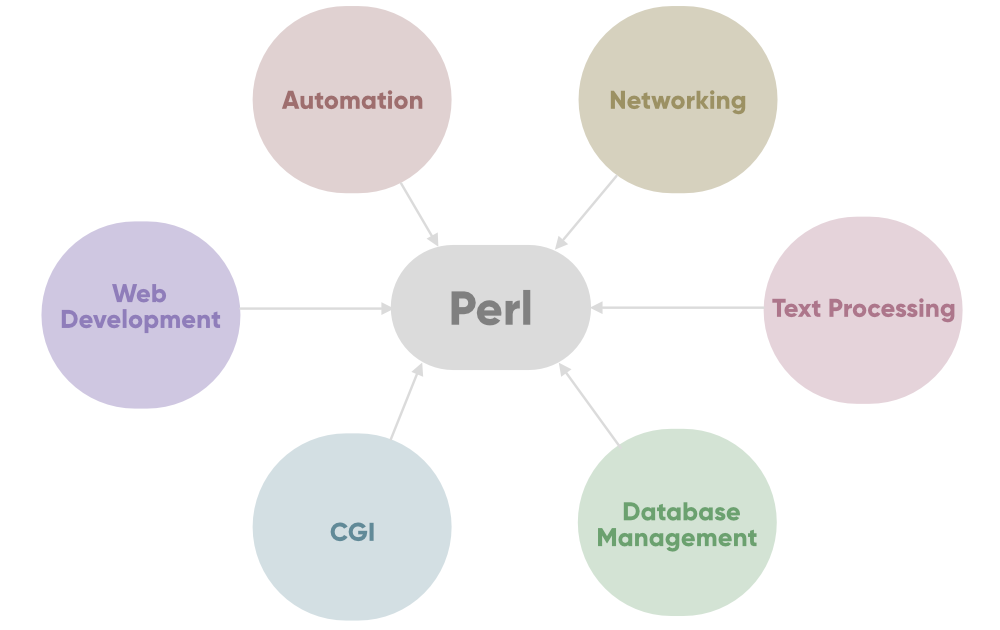### Why and why not use Perl?

WHY USE PERL?WHAT’S WRONG WITH PERL?
Perl Provides supports for cross platform and it is compatible with mark-up languages like HTML, XML etc.Perl doesn’t supports portability due to CPAN modules.
It is free and a Open Source software which is licensed under Artistic and GNU General Public License (GPL).Programs runs slowly and program needs to be interpreted each time when any changes are made.
It is an embeddable language that’s why it can embed in web servers and database servers.In Perl, the same result can be achieved in several different ways which make the code untidy as well as unreadable.
It is very efficient in text-manipulation i.e. Regular Expression. It also provides the socket capability.Usability factor is lower when compared to other languages.

### Getting Started with Perl

Since Perl is a lot similar to other widely used languages syntactically, it is easier to code and learn in Perl. Perl programs can be written on any plain text editor like notepad, notepad++, or anything of that sort. One can also use an online IDE for writing Perl codes or can even install one on their system to make it more feasible to write these codes because IDEs provide a lot of features like intuitive code editor, debugger, compiler, etc.
To begin with, writing Perl Codes and performing various intriguing and useful operations, one must have Perl installed on their System. This can be done by following the step by step instructions provided below:

#### What if Perl already exists?? Let’s check!!!

Many software applications nowadays require Perl to perform their operations, hence a version of Perl might be included in the software’s installation package. Many Linux systems have Perl preinstalled, also Macintosh provides a preinstalled Perl with their Systems.

To check if your device is preinstalled with Perl or not, just go to the Command line(For Windows, search for cmd in the Run dialog( + R), for Linux open the terminal using `Ctrl+Alt+T`, for MacOS use `Control+Option+Shift+T`)
Now run the following command:

`perl -v`

If Perl is already installed, it will generate a message with all the details of the Perl’s version available, otherwise if Perl is not installed then an error will arise stating Bad command or file name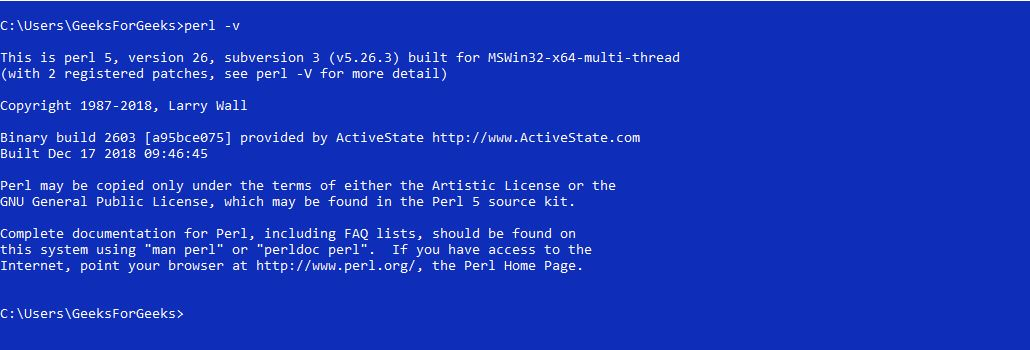Before starting with the installation process, you need to download it. For that, all versions of Perl for Windows, Linux, and MacOS are available on perl.org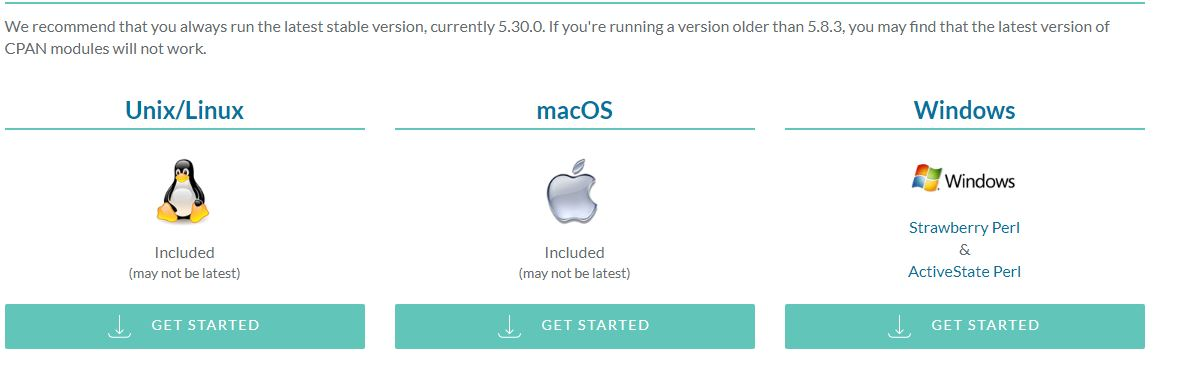Beginning with the Installation:

## Windows

• Getting Started:• Getting done with the User’s License Agreement: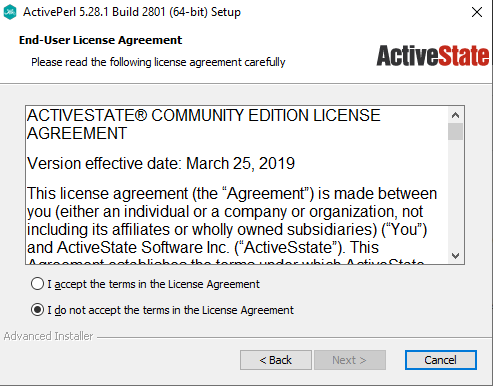• Choosing what to Install: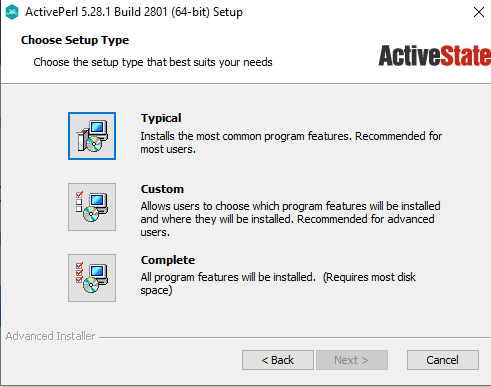• Installation Process: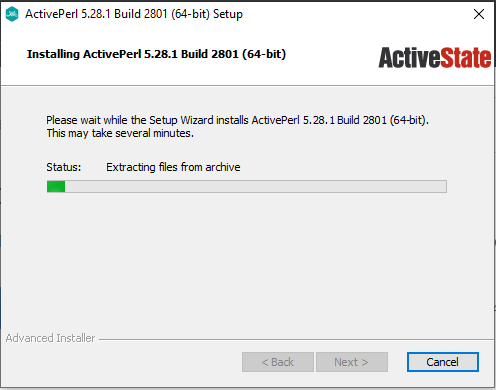• Finished Installation: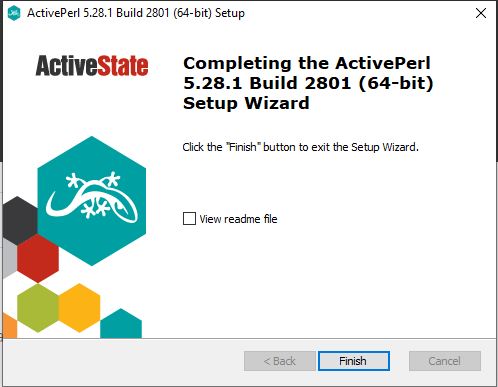## Linux

• Changing Directory to install Perl: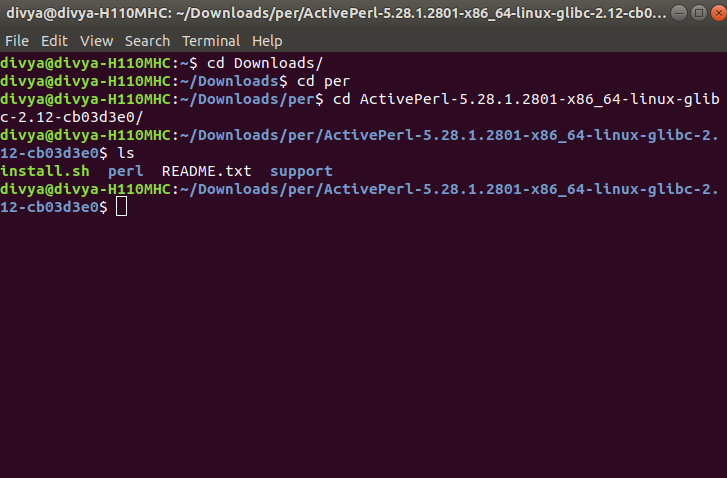• Starting the Installation Process: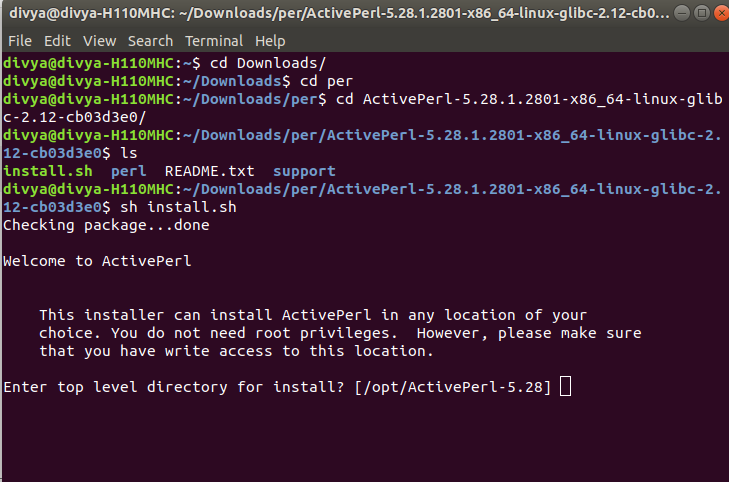• Choosing the Directory to Install Perl: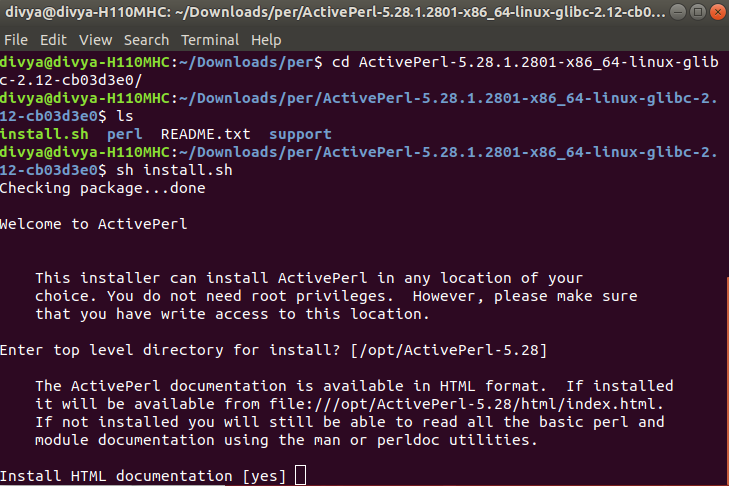• Finishing the Installation: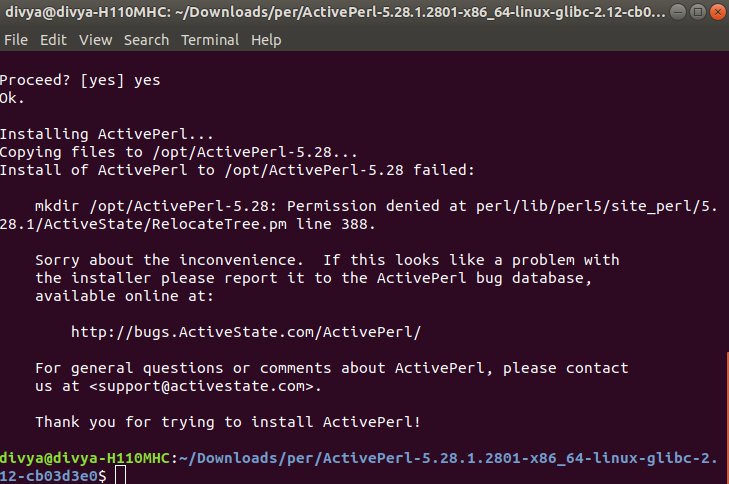## MacOS

• Getting Started: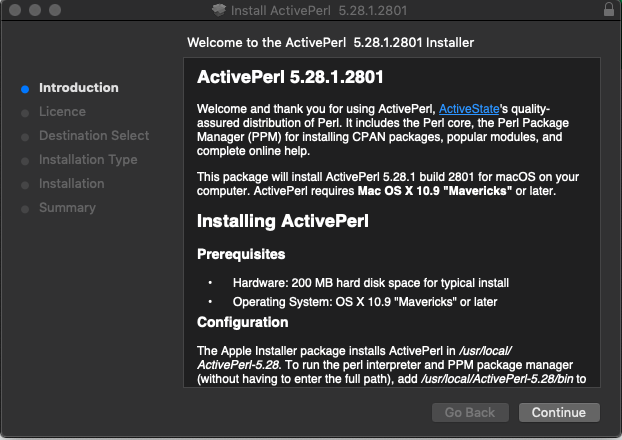• Getting done with the User’s License Agreement: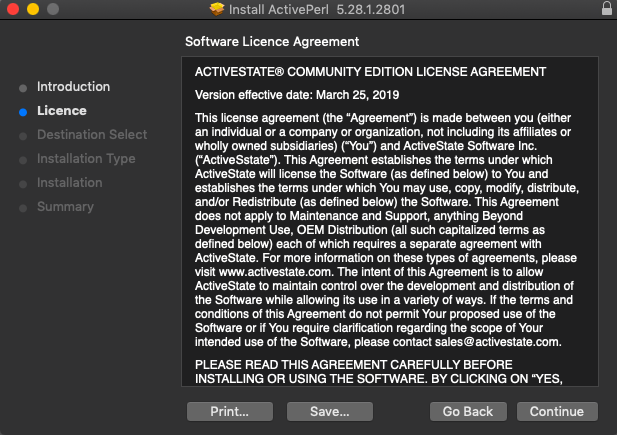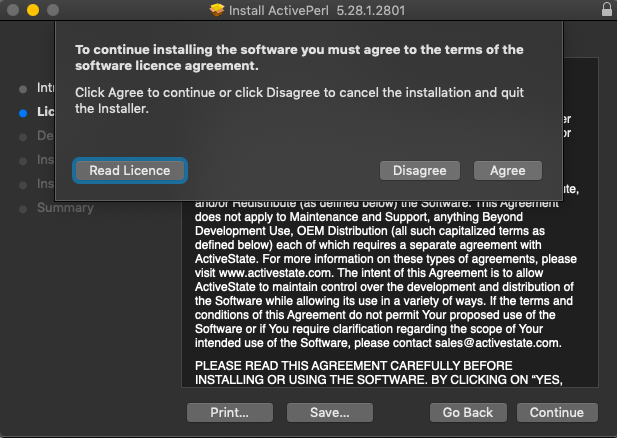• Choosing Installation location: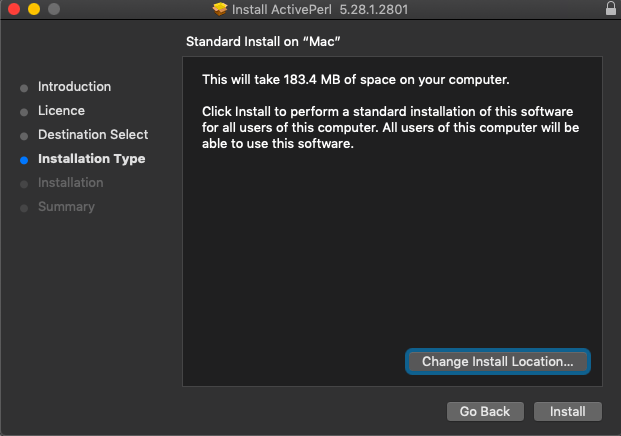• Installation Process: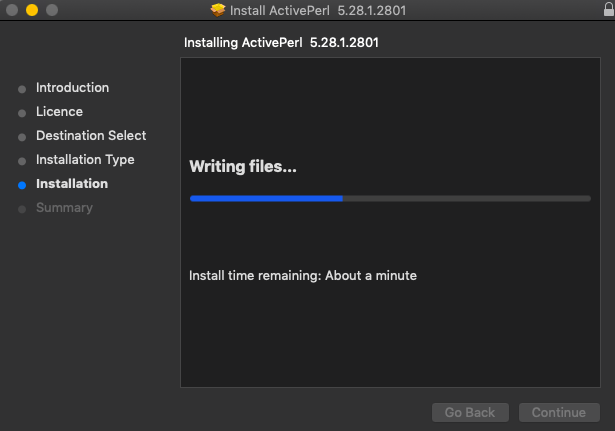• Finishing Installation: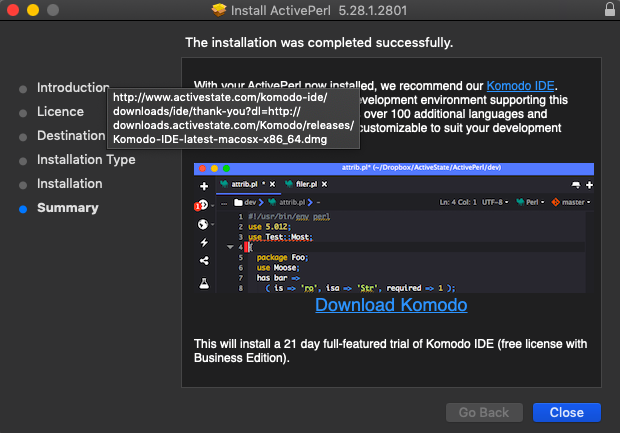#### How to Run a Perl Program?

Let’s consider a simple Hello World Program.

 `#!/usr/bin/perl ``   ` `# Modules used ``use` `strict; ``use` `warnings; ``   ` `# Print function  ``print``(``"Hello World\n"``); `

Generally, there are two ways to Run a Perl program-

• Using Online IDEs: You can use various online IDEs which can be used to run Perl programs without installing.
• Using Command-Line: You can also use command line options to run a Perl program. Below steps demonstrate how to run a Perl program on Command line in Windows/Unix Operating System:

Windows

Open Commandline and then to compile the code type perl HelloWorld.pl. If your code has no error then it will execute properly and output will be displayed.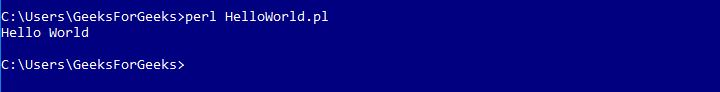Unix/Linux

Open Terminal of your Unix/Linux OS and then to compile the code type perl hello.pl. If your code has no error then it will execute properly and output will be displayed.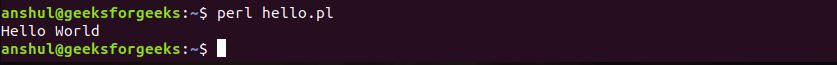## Fundamentals of Perl

#### Variables

Variables are user-defined words that are used to hold the values passed to the program which will be used to evaluate the Code. Every Perl program contains values on which the Code performs its operations. These values can’t be manipulated or stored without the use of a Variable. A value can be processed only if it is stored in a variable, by using the variable’s name.
A value is the data passed to the program to perform manipulation operations. This data can be either numbers, strings, characters, lists, etc.
Example:

```Values:
5
geeks
15

Variables:
\$a = 5;
\$b = "geeks";
\$c = 15;
```

#### Operators

Operators are the main building block of any programming language. Operators allow the programmer to perform different kinds of operations on operands. These operators can be categorized based upon their different functionality:

 `# Perl Program to illustrate the Operators ``   ` `# Operands ``\$a` `= 10; ``\$b` `= 4; ``\$c` `= true;``\$d` `= false;``   ` `# using arithmetic operators  ``print` `"Addition is: "``, ``\$a` `+ ``\$b``, ``"\n"``; ``print` `"Subtraction is: "``, ``\$a` `- ``\$b``, ``"\n"` `; `` ` `# using Relational Operators ``if` `(``\$a` `== ``\$b``) ``{ ``   ``print` `"Equal To Operator is True\n"``; ``}  ``else` `{ ``   ``print` `"Equal To Operator is False\n"``; ``} `` ` `# using Logical Operator 'AND'``\$result` `= ``\$a` `&& ``\$b``; ``print` `"AND Operator: "``, ``\$result``, ``"\n"``; `` ` `# using Bitwise AND Operator ``\$result` `= ``\$a` `& ``\$b``; ``print` `"Bitwise AND: "``, ``\$result``, ``"\n"``; `` ` `# using Assignment Operators ``print` `"Addition Assignment Operator: "``, ``\$a` `+= ``\$b``, ``"\n"``; `

Output:

```Addition is: 14
Subtraction is: 6
Equal To Operator is False
AND Operator: 4
Bitwise AND: 0
```

#### Number and its Types

A Number in Perl is a mathematical object used to count, measure, and perform various mathematical operations. A notational symbol that represents a number is termed a numeral. These numerals, in addition to their use in mathematical operations, are also used for ordering(in the form of serial numbers).

Types of numbers:

• Integers
• Floating Numbers
• Octal Numbers
• Binary Numbers

 `#!/usr/bin/perl`` ` `# Perl program to illustrate``# the use of numbers`` ` `# Integer ``\$a` `= 20; `` ` `# Floating Number ``\$b` `= 20.5647; `` ` `# Scientific value ``\$c` `= 123.5e-10; `` ` `# Hexadecimal Number ``\$d` `= 0xc; `` ` `# Octal Number ``\$e` `= 074; `` ` `# Binary Number ``\$f` `= 0b1010; `` ` `# Printing these values ``print``(``"Integer: "``, ``\$a``, ``"\n"``);``print``(``"Float Number: "``, ``\$b``, ``"\n"``); ``print``(``"Scientific Number: "``, ``\$c``, ``"\n"``); ``print``(``"Hex Number: "``, ``\$d``, ``"\n"``); ``print``(``"Octal number: "``, ``\$e``, ``"\n"``); ``print``(``"Binary Number: "``, ``\$f``, ``"\n"``); `

Output:

```Integer: 20
Float Number: 20.5647
Scientific Number: 1.235e-08
Hex Number: 12
Octal number: 60
Binary Number: 10
```

## DataTypes

Data types specify the type of data that a valid Perl variable can hold. Perl is a loosely typed language. There is no need to specify a type for the data while using it in the Perl program. The Perl interpreter will choose the type based on the context of the data itself.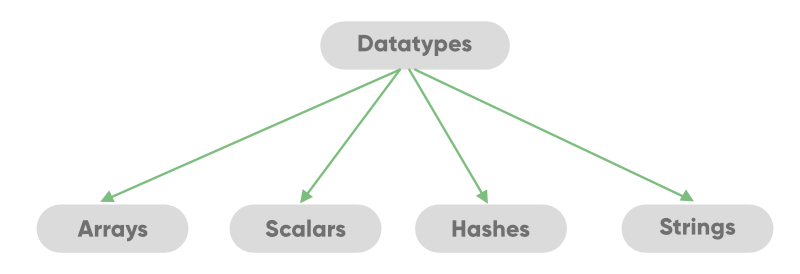#### Scalars

It is a single unit of data which can be an integer number, floating-point, a character, a string, a paragraph, or an entire web page.

Example:

 `# Perl program to demonstrate  ``# scalars variables `` ` `# a string scalar ``\$name` `= ``"Alex"``; ``   ` `# Integer Scalar ``\$rollno` `= 13; ``   ` `# a floating point scalar ``\$percentage` `= 87.65; `` ` `# In hexadecimal form ``\$hexadec` `= 0xcd; `` ` `# Alphanumeric String``\$alphanumeric` `= ``"gfg21"``; `` ` `# special character in string scalar ``\$specialstring` `= ``"^gfg"``; `` ` `# to display the result ``print` `"Name = \$name\n"``; ``print` `"Roll number = \$rollno\n"``; ``print` `"Percentage = \$percentage\n"``; ``print` `"Hexadecimal Form = \$hexadec\n"``; ``print` `"String with alphanumeric values = \$alphanumeric\n"``; ``print` `"String with special characters = \$specialstring\n"``;`

Output:

```Name = Alex
Roll number = 13
Percentage = 87.65
String with alphanumeric values = gfg21
String with special characters = ^gfg
```

#### Arrays

An array is a variable that stores the value of the same data type in the form of a list. To declare an array in Perl, we use ‘@’ sign in front of the variable name.

`@number = (40, 55, 63, 17, 22, 68, 89, 97, 89)`It will create an array of integers that contains the values 40, 55, 63, 17, and many more. To access a single element of an array, we use the ‘\$’ sign.

`\$number`

It will produce the output as 40.

Array creation and accessing elements:

 `#!/usr/bin/perl`` ` `# Perl Program for array creation``# and accessing its elements`` ` `# Define an array``@arr1` `= (1, 2, 3, 4, 5);`` ` `# using qw function ``@arr2` `= ``qw /This ``is a Perl Tutorial by GeeksforGeeks/; `` ` `# Accessing array elements``print` `"Elements of arr1 are:\n"``; ``print` `"\$arr1\n"``;``print` `"\$arr1\n"``;`` ` `# Accessing array elements``# with negative index``print` `"\nElements of arr2 are:\n"``; ``print` `"\$arr2[-1]\n"``;``print` `"\$arr2[-3]\n"``;`

Output:

```Elements of arr1 are:
1
4

Elements of arr2 are:
GeeksforGeeks
Tutorial
```

Iterating through an Array:

 `#!/usr/bin/perl`` ` `# Perl program to illustrate  ``# iteration through an array`` ` `# array creation ``@arr` `= (11, 22, 33, 44, 55);`` ` `# Iterating through the range``print``(``"Iterating through range:\n"``);`` ` `# size of array ``\$len` `= ``@arr``; ``   ` `for` `(``\$b` `= 0; ``\$b` `< ``\$len``; ``\$b` `= ``\$b` `+ 1) ``{ ``    ``print` `"\@arr[\$b] = \$arr[\$b]\n"``; ``} `` ` `# Iterating through loops``print``(``"\nIterating through loops:\n"``);`` ` `# foreach loop ``foreach` `\$a` `(``@arr``) ``{ ``    ``print` `"\$a "``; ``} `

Output:

```Iterating through range:
@arr = 11
@arr = 22
@arr = 33
@arr = 44
@arr = 55

Iterating through loops:
11 22 33 44 55
```

#### Hashes(Associative Arrays)

It is a set of key-value pairs. It is also termed as the Associative Arrays. To declare a hash in Perl, we use the ‘%’ sign. To access the particular value, we use the ‘\$’ symbol which is followed by the key in braces.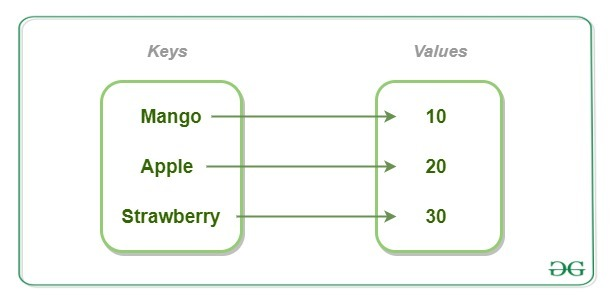Creating and Accessing Hash elements:

 `#!/usr/bin/perl`` ` `# Perl Program for Hash creation``# and accessing its elements`` ` `# Initializing Hash by ``# directly assigning values ``\$Fruit``{``'Mango'``} = 10; ``\$Fruit``{``'Apple'``} = 20; ``\$Fruit``{``'Strawberry'``} = 30; `` ` `# printing elements of Hash``print` `"Printing values of Hash:\n"``; ``print` `"\$Fruit{'Mango'}\n"``; ``print` `"\$Fruit{'Apple'}\n"``; ``print` `"\$Fruit{'Strawberry'}\n"``; `` ` `# Initializing Hash using '=>' ``%Fruit2` `= (``'Mango'` `=> 45, ``'Apple'` `=> 42, ``'Strawberry'` `=> 35); `` ` `# printing elements of Fruit2``print` `"\nPrinting values of Hash:\n"``; ``print` `"\$Fruit2{'Mango'}\n"``; ``print` `"\$Fruit2{'Apple'}\n"``; ``print` `"\$Fruit2{'Strawberry'}\n"``; `

Output:

```Printing values of Hash:
10
20
30

Printing values of Hash:
45
42
35
```

#### Strings

A string in Perl is a scalar variable and starts with a (\$) sign and it can contain alphabets, numbers, special characters. The string can consist of a single word, a group of words, or a multi-line paragraph. The String is defined by the user within a single quote (‘) or double quote (“).

 `#!/usr/bin/perl`` ` `# An array of integers from 1 to 10 ``@list` `= (1..10);  ``   ` `# Non-interpolated string ``\$strng1` `= ``'Using Single quotes: @list'``;  ``   ` `# Interpolated string ``\$strng2` `= ``"Using Double-quotes: @list"``; ``print``(``"\$strng1\n\$strng2"``); `

Output:

```Using Single quotes: @list
Using Double-quotes: 1 2 3 4 5 6 7 8 9 10
```

#### Using Escape character in Strings:

Interpolation creates a problem for strings that contain symbols that might become of no use after interpolation. For example, when an email address is to be stored in a double-quoted string, then the ‘at’ (@) sign is automatically interpolated and is taken to be the beginning of the name of an array and is substituted by it. To overcome this situation, the escape character i.e. the backslash(\) is used. The backslash is inserted just before the ‘@’ as shown below:

 `#!/usr/bin/perl`` ` `# Assigning a variable with an email  ``# address using double-quotes `` ` `# String without an escape sequence ``\$email` `= ``"GeeksforGeeks0402@gmail.com"``;  ``   ` `# Printing the interpolated string ``print``(``"\$email\n"``); ``   ` `# Using '' to escape the  ``# interpolation of '@' ``\$email` `= ``"GeeksforGeeks0402\@gmail.com"``;  ``   ` `# Printing the interpolated string ``print``(``\$email``); `

Output:

```GeeksforGeeks0402.com
GeeksforGeeks0402@gmail.com
```

#### Escaping the escape character:

The backslash is the escape character and is used to make use of escape sequences. When there is a need to insert the escape character in an interpolated string, the same backslash is used, to escape the substitution of escape character with ” (blank). This allows the use of escape characters in the interpolated string.

 `#!/usr/bin/perl ``   ` `# Using Two escape characters to avoid ``# the substitution of escape(\) with blank ``\$string1` `= ``"Using the escape(\\) character"``;  ``   ` `# Printing the Interpolated string ``print``(``\$string1``); `

Output:

```Using the escape(\) character
```

## Control Flow

#### Decision Making

Decision Making in programming is similar to decision-making in real life. A programming language uses control statements to control the flow of execution of the program based on certain conditions. These are used to cause the flow of execution to advance and branch based on changes to the state of a program.

#### Decision Making Statements in Perl :

Example 1: To illustrate use of if and if-else

 `#!/usr/bin/perl`` ` `# Perl program to illustrate ``# Decision-Making statements`` ` `\$a` `= 10;  ``\$b` `= 15;`` ` `# if condition to check ``# for even number ``if``(``\$a` `% 2 == 0 ) ``{  ``    ``printf` `"Even Number"``;  ``}  `` ` `# if-else condition to check ``# for even number or odd number``if``(``\$b` `% 2 == 0 ) ``{  ``    ``printf` `"\nEven Number"``;  ``}  ``else``{    ``    ``printf` `"\nOdd Number"``;  ``} `

Output:

```Even Number
Odd Number
```

Example 2: To illustrate the use of Nested-if

 `#!/usr/bin/perl`` ` `# Perl program to illustrate  ``# Nested if statement ``   ` `\$a` `= 10; ``     ` `if``(``\$a` `% 2 == 0) ``{  ``    ``# Nested - if statement ``    ``# Will only be executed  ``    ``# if above if statement ``    ``# is true ``    ``if``(``\$a` `% 5 == 0) ``    ``{   ``        ``printf` `"Number is divisible by 2 and 5\n"``;   ``    ``}  ``} `

Output:

```Number is divisible by 2 and 5
```

To know more about Decision Making please refer to Decision making in Perl

#### Loops

Looping in programming languages is a feature that facilitates the execution of a set of instructions or functions repeatedly while some condition evaluates to true. Loops make the programmer’s task simpler. Perl provides the different types of loop to handle the condition based situation in the program. The loops in Perl are :

• for loop

 `#!/usr/bin/perl`` ` `# Perl program to illustrate ``# the use of for Loop`` ` `# for loop ``print``(``"For Loop:\n"``);``for` `(``\$count` `= 1 ; ``\$count` `<= 3 ; ``\$count``++) ``{ ``    ``print` `"GeeksForGeeks\n"``}`

Output:

```For Loop:
GeeksForGeeks
GeeksForGeeks
GeeksForGeeks
```
• foreach loop

 `#!/usr/bin/perl`` ` `# Perl program to illustrate ``# the use of foreach Loop`` ` `# Array ``@data` `= (``'GEEKS'``, 4, ``'GEEKS'``); `` ` `# foreach loop ``print``(``"For-each Loop:\n"``);``foreach` `\$word` `(``@data``) ``{ ``    ``print` `(``"\$word "``);``} `

Output:

```For-each Loop:
GEEKS 4 GEEKS
```
• while and do…. while loop

 `#!/usr/bin/perl`` ` `# Perl program to illustrate ``# the use of foreach Loop`` ` `# while loop ``\$count` `= 3; `` ` `print``(``"While Loop:\n"``);``while` `(``\$count` `>= 0) ``{ ``    ``\$count` `= ``\$count` `- 1; ``    ``print` `"GeeksForGeeks\n"``; ``} `` ` `print``(``"\ndo...while Loop:\n"``);``\$a` `= 10; ``   ` `# do..While loop ``do` `{ ``   ` `    ``print` `"\$a "``; ``    ``\$a` `= ``\$a` `- 1; ``} ``while` `(``\$a` `> 0); `

Output:

```While Loop:
GeeksForGeeks
GeeksForGeeks
GeeksForGeeks
GeeksForGeeks

do...while Loop:
10 9 8 7 6 5 4 3 2 1
```

## Object Oriented Programming

Object-oriented programming aims to implement real-world entities like inheritance, hiding, polymorphism, etc in programming. The main aim of OOP is to bind together the data and the functions that operate on them so that no other part of the code can access this data except that function.Creation of a Class and an Object:

 `#!/usr/bin/perl`` ` `# Perl Program for creation of a``# Class and its object``use` `strict; ``use` `warnings; ``   ` `package` `student; ``   ` `# constructor ``sub` `student_data ``{ ``   ` `    ``# shift will take package name 'student'  ``    ``# and assign it to variable 'class' ``    ``my` `\$class_name` `= ``shift``; ``    ``my` `\$self` `= { ``                ``'StudentFirstName'` `=> ``shift``, ``                ``'StudentLastName'` `=> ``shift``               ``}; ``    ``# Using bless function ``    ``bless` `\$self``, ``\$class_name``; ``       ` `    ``# returning object from constructor ``    ``return` `\$self``; ``} ``   ` `# Object creating and constructor calling ``my` `\$Data` `= student_data student(``"Geeks"``, ``"forGeeks"``); ``   ` `# Printing the data ``print` `"\$Data->{'StudentFirstName'}\n"``; ``print` `"\$Data->{'StudentLastName'}\n"``; `

Output:

```Geeks
forGeeks
```

Methods:-
Methods are the entities that are used to access and modify the data of an object. A method is a collection of statements that perform some specific task and returns a result to the caller. A method can perform some specific task without returning anything. Methods are time savers and help us to reuse the code without retyping the code.

 `sub` `Student_data ``{  ``    ``my` `\$self` `= ``shift``;  ``         ` `    ``# Calculating the result  ``    ``my` `\$result` `= ``\$self``->{``'Marks_obtained'``} /  ``                 ``\$self``->{``'Total_marks'``};  ``                      ` `    ``print` `"Marks scored by the student are: \$result"``;  ``}  `

The above-given method can be called again and again wherever required, without doing the effort of retyping the code.

Polymorphism:-
Polymorphism refers to the ability of OOPs programming languages to differentiate between entities with the same name efficiently. This is done by Perl with the help of the signature and declaration of these entities.

Polymorphism can be best explained with the help of the following example:

 `use` `warnings;``  ` `# Creating class using package``package` `A;``sub` `poly_example``{``   ``print``(``"This corresponds to class A\n"``);``}``  ` `package` `B;``sub` `poly_example``{``  ``print``(``"This corresponds to class B\n"``);``}`` ` `B->poly_example();``A->poly_example();`

Output: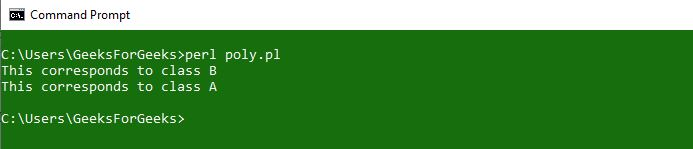Inheritance:-
Inheritance is the ability of any class to extract and use features of other classes. It is the process by which new classes called the derived classes are created from existing classes called Base classes.
Inheritance in Perl can be implemented with the use of packages. Packages are used to create a parent class that can be used in the derived classes to inherit the functionalities.

Encapsulation:-
Encapsulation is the process of wrapping up of data to protect it from the outside sources which need not have access to that part of the code. Technically in encapsulation, the variables or data of a class are hidden from any other class and can be accessed only through any member function of own class in which they are declared. This process is also called Data-Hiding.

Abstraction:-
Abstraction is the process by which the user gets access to only the essential details of a program and the trivial part is hidden from the user. Ex: A car is viewed as a car rather than its individual components. The user can only know what is being done but not the part of How it’s being done. This is what abstraction is.

## Subroutines

#### What are Subroutines?

A Perl function or subroutine is a group of statements that together perform a specific task. In every programming language user want to reuse the code. So the user puts the section of code in function or subroutine so that there will be no need to write code again and again.
Example:

 `#!/usr/bin/perl`` ` `# Perl Program to demonstrate the  ``# subroutine declaration and calling `` ` `# defining subroutine ``sub` `ask_user``{ ``    ``print` `"Hello Geeks!\n"``; ``} ``   ` `# calling subroutine ``# you can also use ``# &ask_user(); ``ask_user(); `

Output:

```Hello Geeks!
```

#### Multiple Subroutines

Multiple subroutines in Perl can be created by using the keyword ‘multi’. This helps in the creation of multiple subroutines with the same name.
Example:

```multi Func1(\$var){statement};
multi Func1(\$var1, \$var2){statement1; statement2;}
```

Example:

 `#!/usr/bin/perl ``# Program to print factorial of a number ``   ` `# Factorial of 0 ``multi Factorial(0) ``{ ``    ``1; ``# returning 1 ``} ``   ` `# Recursive Function  ``# to calculate Factorial ``multi Factorial(Int ``\$n` `where ``\$n` `> 0) ``{ ``    ``\$n` `* Factorial(``\$n` `- 1); ``# Recursive Call ``} ``   ` `# Printing the result  ``# using Function Call ``print` `Factorial(15); `

Output:

`3628800`

To know more about Multiple Subroutines, please refer to Multiple Subroutines in Perl

### Modules and Packages

A module in Perl is a collection of related subroutines and variables that perform a set of programming tasks. Perl Modules are reusable. Perl module is a package defined in a file having the same name as that of the package and having extension .pm. A Perl package is a collection of code which resides in its own namespace.
To import a module, we use require or use functions. To access a function or a variable from a module, :: is used.

Examples:

 `#!/usr/bin/perl`` ` `# Using the Package 'Calculator'``use` `Calculator;`` ` `print` `"Enter two numbers to multiply"``;`` ` `# Defining values to the variables``\$a` `= 5;``\$b` `= 10;`` ` `# Subroutine call``Calculator::multiplication(``\$a``, ``\$b``);`` ` `print` `"\nEnter two numbers to divide"``;`` ` `# Defining values to the variables``\$a` `= 45;``\$b` `= 5;`` ` `# Subroutine call``Calculator::division(``\$a``, ``\$b``);`

Output: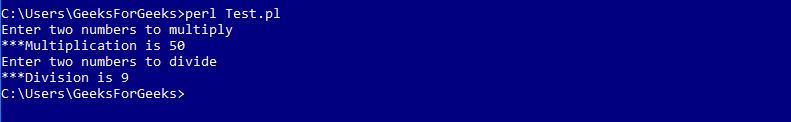#### References

A reference in Perl is a scalar data type that holds the location of another variable. Another variable can be scalar, hashes, arrays, function names, etc. A reference can be created by prefixing it with a backslash.
Example:

 `# Perl program to illustrate the  ``# Referencing and Dereferencing ``# of an Array ``   ` `# defining an array ``@array` `= (``'1'``, ``'2'``, ``'3'``);   ``   ` `# making an reference to an array variable ``\$reference_array` `= \``@array``;   ``   ` `# Dereferencing ``# printing the value stored  ``# at \$reference_array by prefixing  ``# @ as it is a array reference ``print` `@``\$reference_array``;     `

Output:

```123
```

## Regular Expression

Regular Expression (Regex or Regexp or RE) in Perl is a special text string for describing a search pattern within a given text. Regex in Perl is linked to the host language and is not the same as in PHP, Python, etc.
Mostly the binding operators are used with the m// operator so that the required pattern could be matched out.

Example:

 `# Perl program to demonstrate ``# the m// and =~ operators ``   ` `# Actual String ``\$a` `= ``"GEEKSFORGEEKS"``; ``   ` `# Prints match found if  ``# its found in \$a ``if` `(``\$a` `=~ m[GEEKS])  ``{ ``    ``print` `"Match Found\n"``; ``} ``   ` `# Prints match not found  ``# if its not found in \$a ``else` `{ ``    ``print` `"Match Not Found\n"``; ``} `

Output:

```Match Found
```

Output:

`Match Found`

#### Quantifiers in Regex

Perl provides several numbers of regular expression quantifiers which are used to specify how many times a given character can be repeated before matching is done. This is mainly used when the number of characters going to be matched is unknown.
There are six types of Perl quantifiers which are given below:

• * = This says the component must be present either zero or more times.
• + = This says the component must be present either one or more times.
• ? = This says the component must be present either zero or one time.
• {n} = This says the component must be present n times.
• {n, } = This says the component must be present at least n times.
• {n, m} = This says the component must be present at least n times and no more than m times.

## File Handling

In Perl, a FileHandle associates a name to an external file, that can be used until the end of the program or until the FileHandle is closed. In short, a FileHandle is like a connection that can be used to modify the contents of an external file and a name is given to the connection (the FileHandle) for faster access and ease.
The three basic FileHandles in Perl are STDIN, STDOUT, and STDERR, which represent Standard Input, Standard Output, and Standard Error devices respectively.

#### Reading from and Writing to a File using FileHandle

Reading from a FileHandle can be done through the print function.

 `# Opening the file  ``open``(fh, ``"GFG2.txt"``) or ``die` `"File '\$filename' can't be opened"``; ``   ` `# Reading First line from the file ``\$firstline` `= ; ``print` `"\$firstline\n"``; `

Output :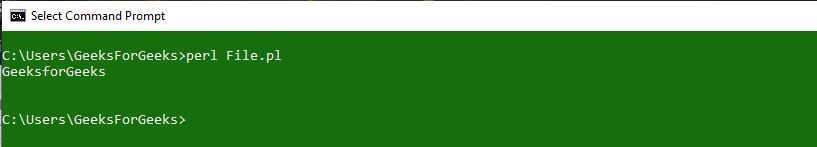Writing to a File can also be done through the print function.

 `# Opening file Hello.txt in write mode``open` `(fh, ``">"``, ``"Hello.txt"``);`` ` `# Getting the string to be written``# to the file from the user``print` `"Enter the content to be added\n"``;``\$a` `= <>;`` ` `# Writing to the file``print` `fh ``\$a``;`` ` `# Closing the file``close``(fh) or ``"Couldn't close the file"``; `

Executing Code to Write: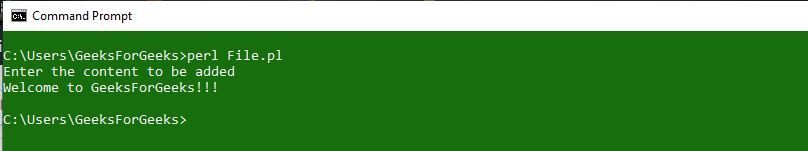Updated File: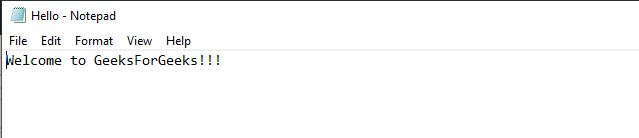Basic Operations on Files
Multiple Operations can be performed on files using FileHandles. These are:

#### File Test Operators

File Test Operators in Perl are the logical operators that return True or False values. There are many operators in Perl that you can use to test various different aspects of a file. For example, to check for the existence of a file -e operator is used.
Following example uses the ‘-e’, existence operator to check if a file exists or not:

 `#!/usr/bin/perl`` ` `# Using predefined modules``use` `warnings;``use` `strict;``  ` `# Providing path of file to a variable``my` `\$filename` `= ``'C:\Users\GeeksForGeeks\GFG.txt'``;`` ` `# Checking for the file existence``if``(-e ``\$filename``)``{``     ` `    ``# If File exists``    ``print``(``"File \$filename exists\n"``);``}`` ` `else``{``     ` `    ``# If File doesn't exists``    ``print``(``"File \$filename does not exists\n"``);``}`

Output: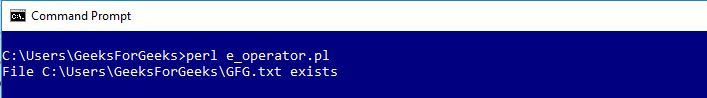To know more about various different Operators in File Testing, please refer to File Test Operators in Perl

#### Working with Excel Files

Excel files are the most commonly used office application to communicate with computers. For creating excel files with Perl you can use padre IDE, we will also use Excel::Writer::XLSX module.
Perl uses write() function to add content to the excel file.

Creating an Excel File:
Excel Files can be created using Perl command line but first we need to load Excel::Writer::XLSX module.

 `#!/usr/bin/perl ``use` `Excel::Writer::XLSX;`` ` `my` `\$Excelbook` `= Excel::Writer::XLSX->new( ``'GFG_Sample.xlsx'` `);``my` `\$Excelsheet` `= ``\$Excelbook``->add_worksheet();`` ` `\$Excelsheet``->``write``( ``"A1"``, ``"Hello!"` `);``\$Excelsheet``->``write``( ``"A2"``, ``"GeeksForGeeks"` `);``\$Excelsheet``->``write``( ``"B1"``, ``"Next_Column"` `);`` ` `\$Excelbook``->``close``;`

Output: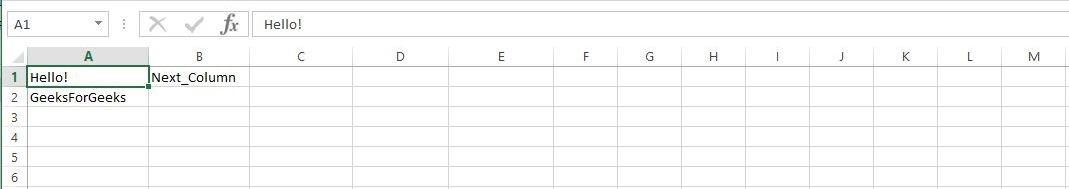Reading of an Excel File in Perl is done by using `Spreadsheet::Read` module in a Perl script. This module exports a number of function that you either import or use in your Perl code script. `ReadData()` function is used to read from an excel file.
Example:

 `use` `5.016;``use` `Spreadsheet::Read ``qw(ReadData)``;``my` `\$book_data` `= ReadData (‘new_excel.xlsx');``say` `'A2: '` `. ``\$book_data``->{A2};`

Output:

`A2: GeeksForGeeks`

## Error Handling

Error Handling in Perl is the process of taking appropriate action against a program that causes difficulty in execution because of some error in the code or the compiler. For example, if opening a file that does not exist raises an error, or accessing a variable that has not been declared raises an error.
The program will halt if an error occurs, and thus using error handling we can take appropriate action instead of halting a program entirely. Error Handling is a major requirement for any language that is prone to errors.

Perl provides two built-in functions to generate fatal exceptions and warnings, that are:

1. die()
2. warn()

die(): To signal occurrences of fatal errors in the sense that the program in question should not be allowed to continue.
For example, accessing a file with `open()` tells if the open operation is successful before proceeding to other file operations.

`open FILE, "filename.txt" or die "Cannot open file: \$!\n";`

warn(): Unlike `die()` function, `warn()` generates a warning instead of a fatal exception.

For example:

`open FILE, "filename.txt" or warn "Cannot open file: \$!\n";`

To know more about various different Error Handling techniques, please refer to Error Handling in Perl

My Personal Notes arrow_drop_up